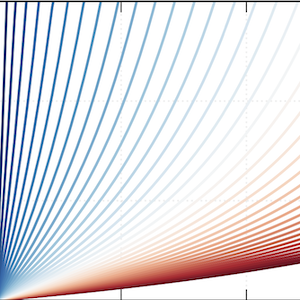## Modeling the Effects of Salt Concentration on Aqueous and Organic Electrolytes

van der Lubbe S., and Canepa P.; ChemRxiv, (2023).

## Abstract

Understanding the thermodynamic properties of electrolyte solutions is of vital importance for a myriad of physiological and technological applications. The activity coefficient $\gamma$$\pm$ is associated with the deviation of an electrolyte solution from its ideal behavior and may be obtained by combining the Debye-Hückel (DH) and Born (B) equations. However, the DH and B equations depend on the concentration and temperature-dependent static permittivity of the solution $\epsilon$r (c, T) and size of the solvated ions r$_i$, whose experimental data is often not available. In this work, we use a combination of molecular dynamics and density functional theory to predict $\epsilon$r (c, T) and r$_i$, which enables us to apply the DH and B equations to any technologically relevant electrolyte at any concentration and temperature of interest.